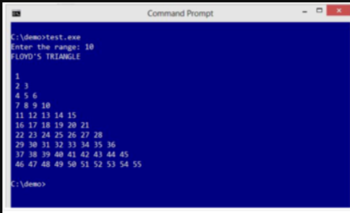# C Program to find sum of two numbersC Program to find sum of two numbers

#include<stdio.h>

int main()

{

int a, b, sum;

printf("\nEnter two no: ");

scanf("%d %d", &a, &b);

sum = a + b;

printf("Sum : %d", sum);

return(0);

}

Output :

Enter two no: 5 6

Sum : 11